# What is the effective capacitance between points X and Y?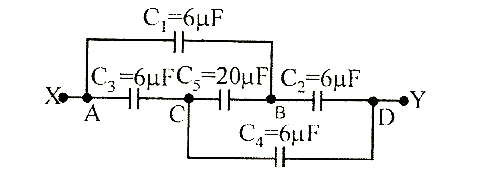(a)    24 mF         (b)    18 mF         (c)    12 mF         (d)    6 mFAditi Chauhan
10 years ago

(d)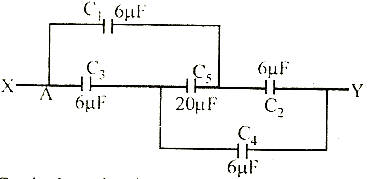Equivalent circuit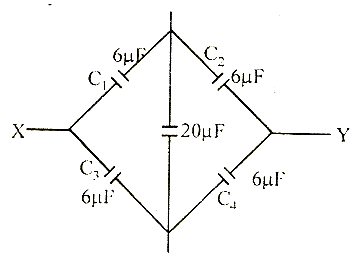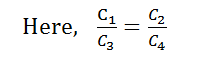Hence, no charge will flow through 20 mF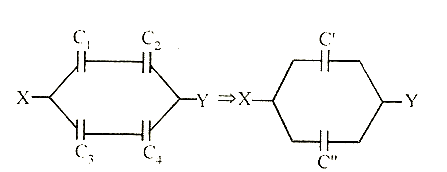C1 and C2 are in series, also C3 and C4 are in series.

Hence, C'' = 3 mF, C" = 3 mF

C'' and C" are in parallel.

Hence net capacitance = C'' + C" = 3 + 3 = 6 mF

3 years ago
Dear Student,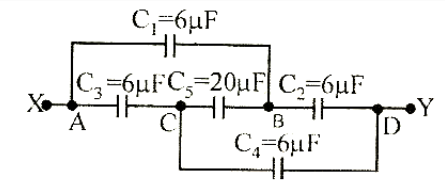Equivalent circuit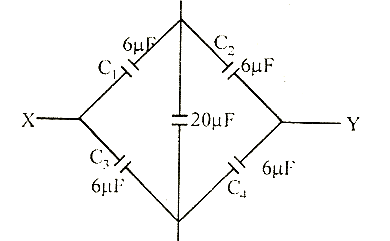Hence
C1/C3 = C2/C4
Hence, no charge will flow through 20 mFC1 and C2 are in series, also C3 and C4 are in series.
Hence, C'' = 3 mF, C" = 3 mF
C'' and C" are in parallel.
Hence net capacitance = C'' + C" = 3 + 3 = 6 mF

Thanks and Regards